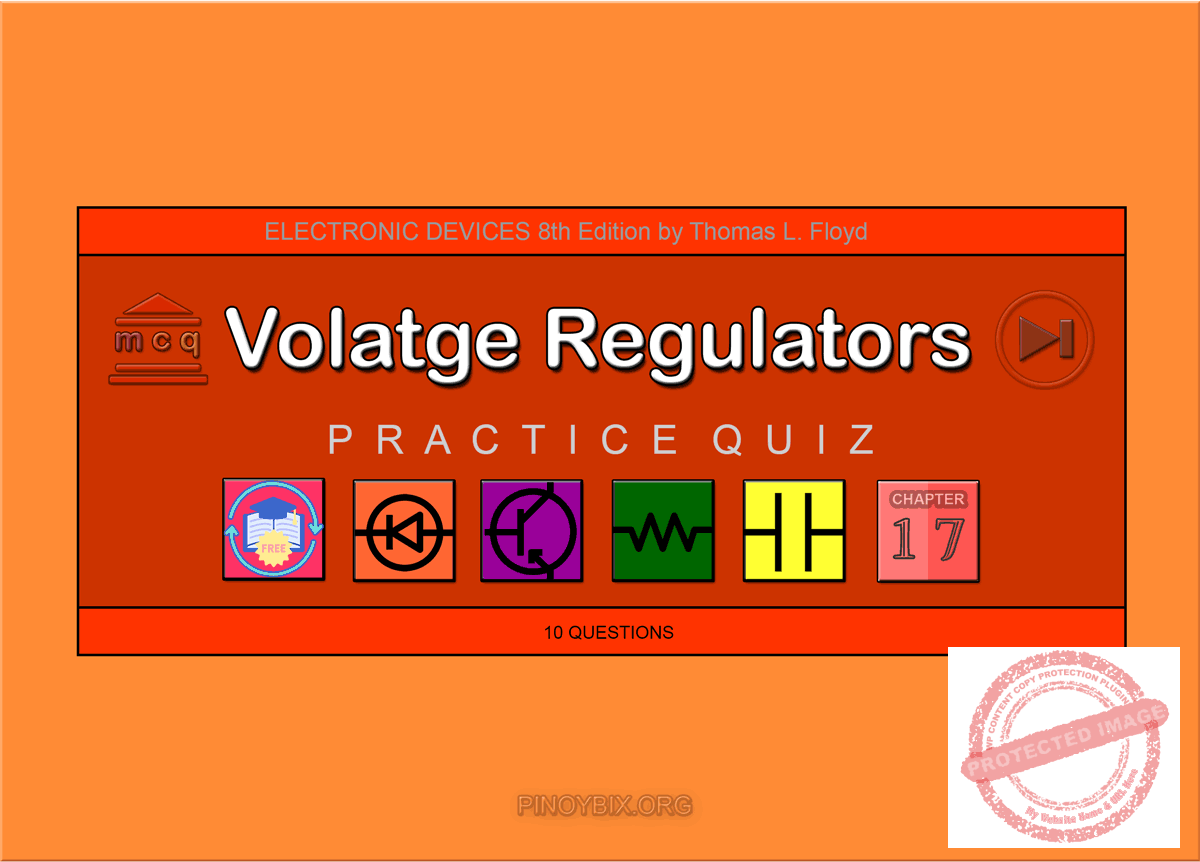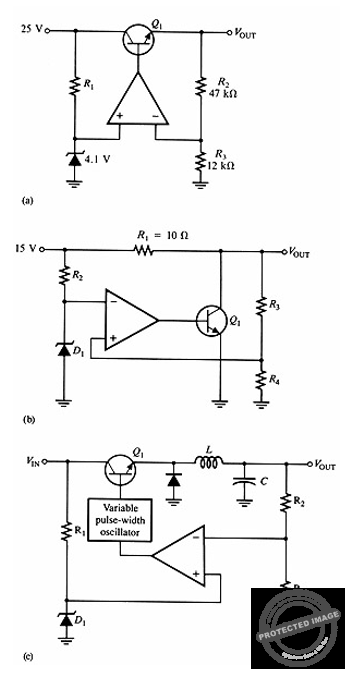# Floyd: MCQ in Voltage Regulators

(Last Updated On: December 9, 2019)This is the Multiple Choice Questions in Voltage Regulators from the book Electronic Devices – Electron Flow Version and Conventional Current Version 8th Edition by Thomas L. Floyd. If you are looking for a reviewer in Electronics Engineering this will definitely help. I can assure you that this will be a great help in reviewing the book in preparation for your Board Exam. Make sure to familiarize each and every questions to increase the chance of passing the ECE Board Exam.

If you are looking for the Self-test in Floyd’s Electronic Devices proceed to

#### Practice Exam Test Questions

Choose the letter of the best answer in each questions.

1. Voltage regulation requires

A. only line regulation.

Solution:

2. In Figure 17-1(a)(see question 6), the purpose of R1 is to

A. provide a reference voltage.

B. limit current through the diode.

C. limit voltage to the op-amp.

D. provide output voltage sampling.

Solution:

3. What type of regulator offers inherent short-circuit protection?

A. shunt regulators

B. series regulators

C. three-terminal regulators

D. switching regulators

Solution:

4. In a series regulator, what is the purpose of fold-back limiting?

A. to provide more current in the case of a short circuit

B. to limit output voltage if input voltage goes too high

C. to bypass the pass-transistor, if the pass-transistor should fail

D. to provide current up to a maximum, but drop current to a lower value when the output becomes shorted, to prevent overheating of the device

Solution:

5. A switching regulator that is configured as a voltage-inverter produces what type of output?

A. an ac output with opposite phase to the input ac

B. a dc output that is the negative of the dc input voltage

C. an output that is a higher voltage than the input voltage

D. an ac output from a dc input voltage

Solution:

6. Refer to Figure 17-1(a). Determine the output voltage, VOUT.Figure 17-1

A. 25 V

B. 5.1 V

C. 20.2 V

D. 4.1 V

Solution:

7. Refer to Figure 17-1(b). If the load is short-circuited, what would be the maximum current through R1?

A. 1 A

B. 1.2 A

C. 1.5 A

D. 5 A

Solution:

8. Refer to Figure 17-1(c). This circuit is called

A. a series-pass voltage regulator.

B. a shunt voltage regulator.

C. a step-up switching regulator.

D. a step-down switching regulator.

Solution:

9. Refer to Figure 17-1(b). This circuit is known as

A. a series-pass voltage regulator.

B. a shunt voltage regulator.

C. a step-up switching regulator.

D. a step-down switching regulator.

Solution:

10. Refer to Figure 17-1(a). This circuit is known as

A. a series-pass voltage regulator.

B. a shunt voltage regulator.

C. a step-up switching regulator.

D. a step-down switching regulator.

Solution:

11. Refer to Figure 17-1(c). This circuit operates at a _____ frequency, and its efficiency is _____.

A. low, low

B. low, high

C. high, high

D. high, low

Solution:

12. Refer to Figure 17-1(c). The inductor and capacitor are used for

A. amplifying the error signal.

C. turning on the pulse-width oscillator.

D. filtering the dc pulse output.

Solution:

13. Refer to Figure 17-1(b). The purpose for the zener diode D1 is

A. to supply a reference voltage.

B. to amplify the error signal.

C. to sense the error signal.

D. to limit the input voltage to the circuit.

Solution:

14. A voltage regulator has a no-load output of 18 V and a full-load output of 17.3 V. The percent load regulation is

A. 0.25%.

B. 96.1%.

C. 4.05%.

D. 1.04%.

Solution:

15. A voltage regulator with a no-load output dc voltage of 12 V is connected to a load with a resistance of 10 Ω. If the load resistance decreases to 7.5 Ω, the load voltage will decrease to 10.9 V. The load current will be _____, and the percent load regulation is _____.

A. 1.45 A, 90.8%

B. 1.45 A, 10.09%

C. 1.2 A, 90.8%

D. 1.2 A, 10.09%

Solution:

16. Refer to Figure 17-1(b). If the output of the circuit were to be a short circuit, what power rating would R1 need to have?

A. 2.25 W

B. 5 W

C. 10 W

D. 22.5 W

Solution:

17. Refer to Figure 17-1(a). If the zener diode had a rating of 1.7 V, the output voltage would be

A. 2.13 V.

B. 8.36 V.

C. 20.16 V.

D. 25 V.

Solution:

18. Refer to Figure 17-1(a). If the BE junction of Q1 opens, the output voltage VOUT will

A. increase.

B. decrease.

C. remain the same.

D. none of the above

Solution:

19. Refer to Figure 17-1(a). If a solder splash shorted the ends of R1 to each other,

A. the op-amp would fail.

B. Q1 would open.

C. the output voltage would not change.

D. the zener would fail.

Solution:

20. Refer to Figure 17-1(c). If the output voltage tends to decrease due to an increase in load current, the transistor will conduct for _______ time each cycle.

A. a longer

B. a shorter

C. the same

D. none of the above

Solution:

21. Voltage regulators keep a constant _____ output voltage when the input or load varies within limits.

A. dc

B. ac

C. ripple

D. none of the above

Solution:

22. _____ regulation can be defined as the percentage change in the output voltage for a given change in the input voltage.

A. Line

C. any of the above

D. none of the above

Solution:

23. _____ regulation can be defined as the percentage change in the output voltage for a given change in load current.

A. Line

C. any of the above

D. none of the above

Solution:

24. In a series linear regulator, the control element is a(n) _____ in series with the load.

A. inductor

B. capacitor

C. transistor

D. none of the above

Solution:

25. In a shunt linear regulator, the control element is a(n) _____ in parallel with the load.

A. inductor

B. capacitor

C. transistor

D. none of the above

Solution:

26. The _____ regulator is less efficient than the _____ type, but offers inherent short-circuit protection.

A. series, shunt

B. shunt, series

C. any of the above

D. none of the above

Solution:

27. Switching regulators are more efficient than linear regulators and are particularly useful in _____-voltage, _____-current applications.

A. high, high

B. low, high

C. high, low

D. none of the above

Solution:

28. Switching regulator efficiencies can be greater than _____ percent.

A. 60

B. 70

C. 80

D. 90

Solution:

29. How many terminals does a 7800 series IC regulator have?

A. 2

B. 3

C. 4

D. none of the above

Solution:

#### TRUE/FALSE

1. A basic voltage regulator consists largely of a reference source, an error detector, and a control device.

A. True

B. False

Solution:

2. Switching regulators are not very efficient.

A. True

B. False

Solution:

3. IC voltage regulators are sometimes called three-terminal regulators.

A. True

B. False

Solution:

4. Load regulation is the percentage change in line voltage for a change in load voltage.

A. True

B. False

Solution:

5. A pass transistor may be used in a series regulator, as the current-carrying device.

A. True

B. False

Solution:

6. Line regulation is the percentage change in output voltage for a given change in input line current.

A. True

B. False

Solution:

7. Under no-load, a shunt regulator has minimum current through the control element.

A. True

B. False

Solution:

8. A switching regulator can be configured as a step-up or a step-down voltage regulator.

A. True

B. False

Solution:

9. For an IC regulator, the input voltage must be at least 2 V above the regulated output voltage.

A. True

B. False

Solution:

10. A three-terminal regulator can be used with an external pass transistor to increase the total load current available.

A. True

B. False

Solution:

### Complete List of Chapter MCQ in Floyd’s Electronic Devices

P inoyBIX educates thousands of reviewers and students a day in preparation for their board examinations. Also provides professionals with materials for their lectures and practice exams. Help me go forward with the same spirit.

“Will you subscribe today via YOUTUBE?”

Subscribe

PinoyBIX Engineering. © 2014-2020 All Rights Reserved | How to Donate? |